# 搞懂 Vision Transformer 原理和代码，看这篇技术综述就够了（六）

## 本文目录

13 CvT: Introducing Convolutions to Vision Transformers
(来自麦吉尔大学, 微软云+AI)
13.1 CvT原理分析

14 CeiT：将卷积设计整合到视觉Transformers中
(来自商汤)
14.1 CeiT原理分析

Transformer 是 Google 的团队在 2017 年提出的一种 NLP 经典模型，现在比较火热的 Bert 也是基于 Transformer。Transformer 模型使用了 Self-Attention 机制，不采用 RNN 的顺序结构，使得模型可以并行化训练，而且能够拥有全局信息。

## 13 CvT: Introducing Convolutions to Vision Transformers

https://arxiv.org/pdf/2103.15808.pdf​arxiv.org

• 13.1 CvT原理分析：

Motivation

Transformer已经被证明在许多视觉任务上可以取得不错的性能。ViT是首创的把Transformer应用在分类任务的工作。但是，ViT的性能弱于具有相似的尺寸的CNN的性能，而且，ViT所需要的训练数据量要比同尺寸的CNN模型大很多。作者认为这是因为基于Transformer的ViT缺乏了一些CNN特有的 "desirable properties" ，而这些properties使得CNN模型很适合视觉任务。这句话应该如何理解呢？请参考我的下面这个回答：

CNN是不是一种局部self-attention？​www.zhihu.com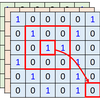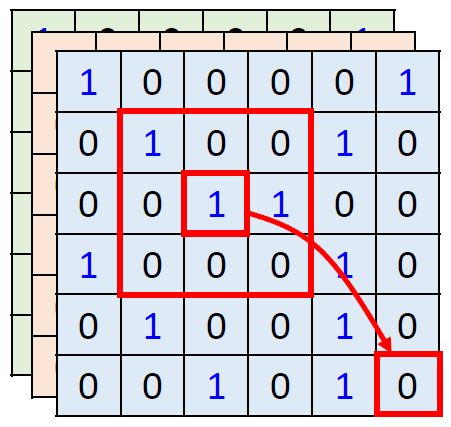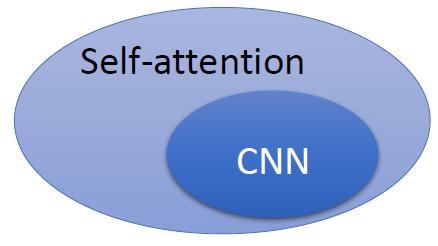CvT具有CNN的一些优良的特征：局部感受野，共享卷积权重，空间下采样。

CvT具有Self-attention的一些优良的特征：动态的注意力机制，全局信息的融合。

CvT具体的结构如下图3所示。总的pipeline是一个multi-stage的hierarchical的结构。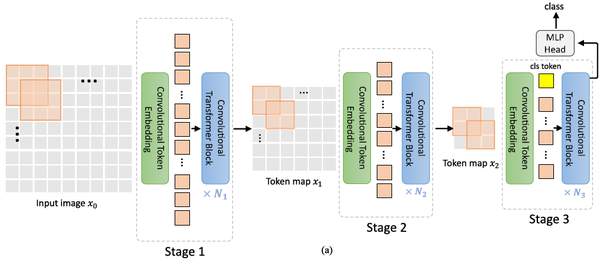Convolutional Token Embedding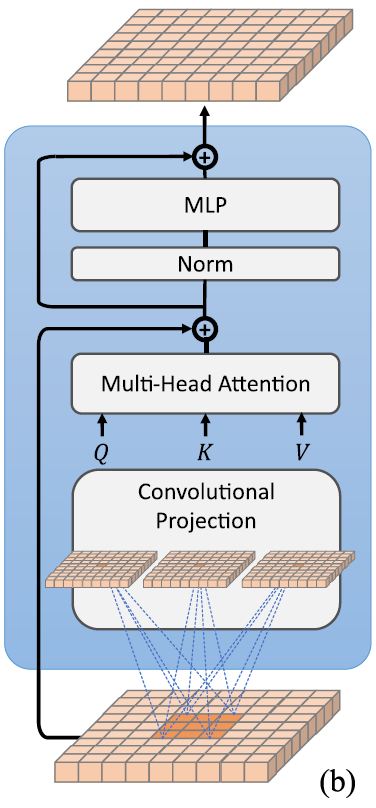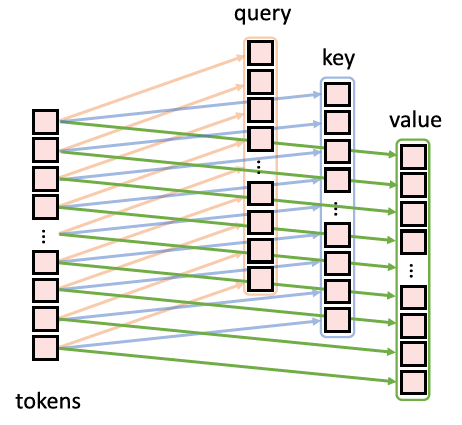$$\begin{equation} x_i^{q/k/v} = \texttt{Flatten}\left(\texttt{Conv2d}\left(\texttt{Reshape2D}(x_i), \; s\right)\right), \end{equation} \tag{13.1}$$

$\texttt{Depth-wise Conv2d} \rightarrow \texttt{BatchNorm2d} \rightarrow\texttt{Point-wise Conv2d}$ 。 $(k=s)$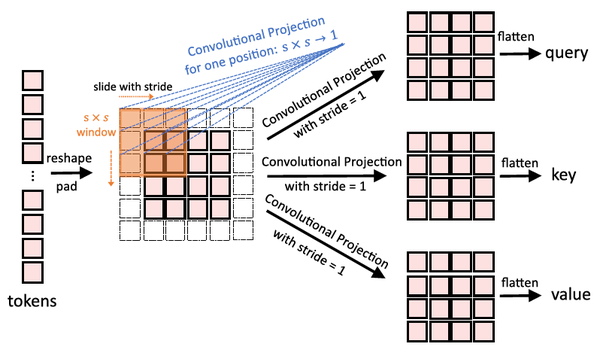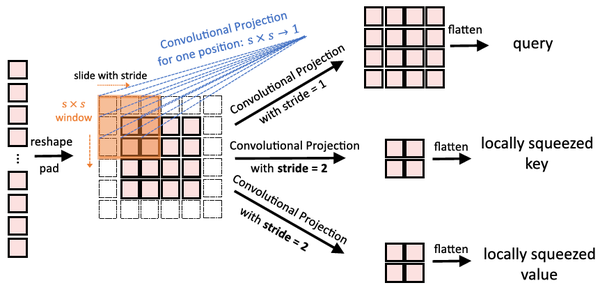• 不再使用位置编码。
• class token只加在最后一个stage里面。

Experiments：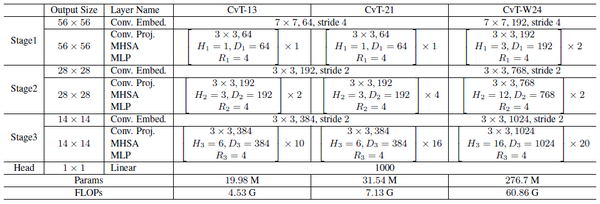Fine-tuning： 微调的策略与ViT一致，优化器使用SGD，momentem取0.9。Fine-tune的办法是：都首先在分辨率224×224的数据上进行训练，后面在384×384的数据上finetune。batch size = 512，fine-tune的数据集有ImageNet-1k，CIFAR-10，CIFAR-100，Oxford-IIIT Pets，Oxford-IIIT Flowers-102。

CvT模型可以以较低的参数量和计算量达到更好的性能，比如21层的CvT在ImageNet上可以达到82.5\%的高性能，比DeiT-B的性能还要好，而参数量和计算量都有大幅地下降。

CvT模型也展示出了比最近刚出的新模型还要更优的性能：比如在相当的参数量和计算量的前提下性能优于TNT，T2T，PVT-small等等。

CvT系列最大的模型：CvT-W24可以在ImageNet上达到87.7\%的性能，不需要JFT-300预训练，超过了ViT-L模型。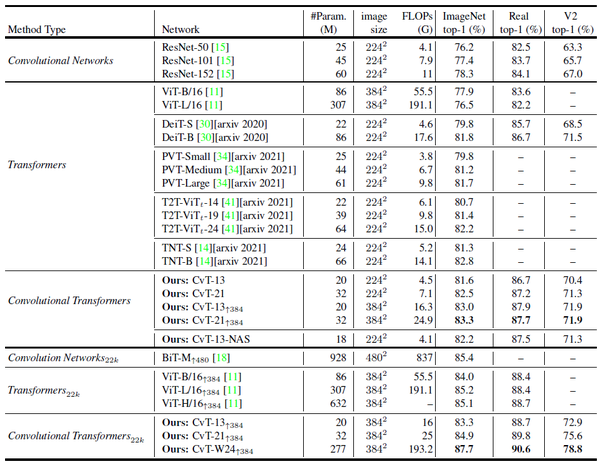CvT在小数据集上的结果如图10所示。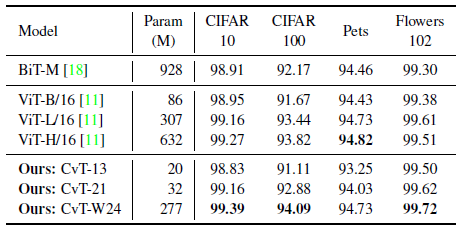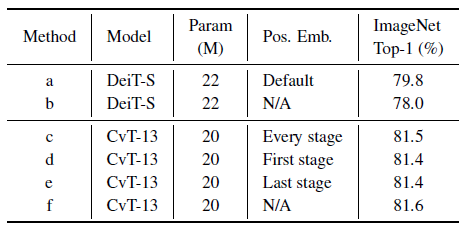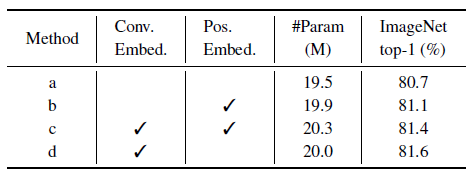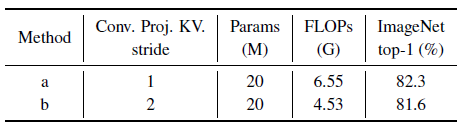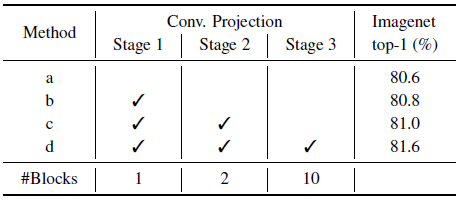CvT是如何将卷积操作引入Transformer的呢？

CvT模型算是把模型从Transformer向着CNN的方向又拉了一把。

## 14 CeiT：将卷积设计整合到视觉Transformers中

https://arxiv.org/pdf/2103.11816.pdf​arxiv.org

• 14.1 CeiT原理分析：

CeiT也是想借助CNN来提升Transformer的性能，作者给出的理由与CvT大致思路一致，二者几乎是同时提出的，所以解释这个问题的思路一致也很正常。作者认为CNN的最重要的特征是不变性 (invariance)局部性 (locality)

1. 为了有效地提取low-level feature，作者通过 Image-to-tokens 先使用卷积+Flatten操作把图片变为tokens，而不是通过直接分patch的方法。
2. 为了强化局部特征的提取，作者把MLP层的Feed-Forwardnetwork 换成了 Locally-enhanced Feed-Forward layer，在空间维度上促进相邻token之间的相关性。
3. 除此之外，在Transformer顶部使用 Layer-wise Class token Attention 进一步提升性能。

Image-to-tokens 模块

ViT采用的是直接把一张 $H\times W$ 的图片分成 $N$ 个patch，每个patch的大小是 $P\times P$ 的，所以patch的数量 $N=HW/P^2$ 。但如上文所述，这么做会很难捕捉到low-level的信息，比如图片的边和角的信息。而且，self-attention建模的是全局的信息，所以相当于是使用了很大的kernel，这样的kernel由于参数量过多导致很难训练，需要大量的数据。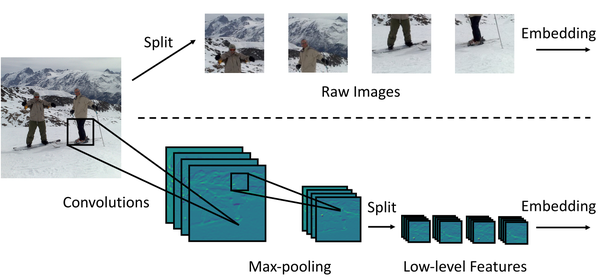$$\begin{equation} \mathbf{x}^{\prime} = \mbox{I2T}(\mathbf{x}) = \mbox{MaxPool}(\mbox{BN}(\mbox{Conv}(\mathbf{x}))) \end{equation} \tag{16.1}$$

I2T模块充分利用了 $CNN$ 在提取low-level的特征方面的优势，通过缩小patch的大小来降低嵌入的训练难度。

Locally-enhanced Feed-Forward layer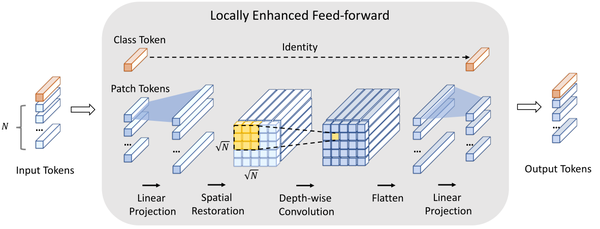LeFF的具体流程是：首先，输入的token $\mathbf{x}_t^{h}\in \mathbb{R}^{(N+1)\times C}$ 由前面的MSA模块得到，然后分成2部分，第1部分是把class token $\mathbf{x}_c^{h}\in \mathbb{R}^{C}$ 单独拿出来，剩下的第2部分是 $\mathbf{x}_p^{h}\in \mathbb{R}^{N\times C}$ 。接着把第2部分通过linear projection把 $\mathbf{x}_p^{h}\in \mathbb{R}^{N\times C}$ 拓展到高维的 $\mathbf{x}_p^{l_1}\in \mathbb{R}^{N\times (e\times C)}$ ，其中 $e$ 代表expand ratio。接着将其还原成2D的图片 $\mathbf{x}_p^{s}\in \mathbb{R}^{\sqrt{N}\times \sqrt{N}\times (e\times C)}$ ，再通过Depth-wise convolution得到 $\mathbf{x}_p^{d}\in \mathbb{R}^{\sqrt{N}\times \sqrt{N}\times (e\times C)}$ ，再Flatten成 $\mathbf{x}_p^{f}\in \mathbb{R}^{N\times (e\times C)}$ 的张量。最后通过Linear Projection映射回原来的维度 $\mathbf{x}_p^{l_2}\in \mathbb{R}^{N\times C}$ ，并与一开始的class token concat起来得到 $\mathbf{x}_t^{h+1}\in \mathbb{R}^{(N+1)\times C}$ 。每个Linear Projection和convolution之后都会加上BatchNorm 和 GELU 操作。总的流程可以写成下式：

\begin{align} \mathbf{x}_c^h, \mathbf{x}_p^h &= \mbox{Split}(\mathbf{x}_t^h) \ \mathbf{x}_p^{l_1} &= \mbox{GELU}(\mbox{BN}(\mbox{Linear1}(\mathbf{x}_p^h))) \ \mathbf{x}_p^{s} &= \mbox{SpatialRestore}(\mathbf{x}_p^{l_1}) \ \mathbf{x}_p^{d} &= \mbox{GELU}(\mbox{BN}(\mbox{DWConv}(\mathbf{x}_p^{s}))) \ \mathbf{x}_p^{f} &= \mbox{Flatten}(\mathbf{x}_p^{d}) \ \mathbf{x}_p^{l_2} &= \mbox{GELU}(\mbox{BN}(\mbox{Linear2}(\mathbf{x}_p^f))) \ \mathbf{x}_t^{h+1} &= \mbox{Concat}(\mathbf{x}_c^h, \mathbf{x}_p^{l_2}) \end{align} \tag{16.2}

Layer-wise Class token Attention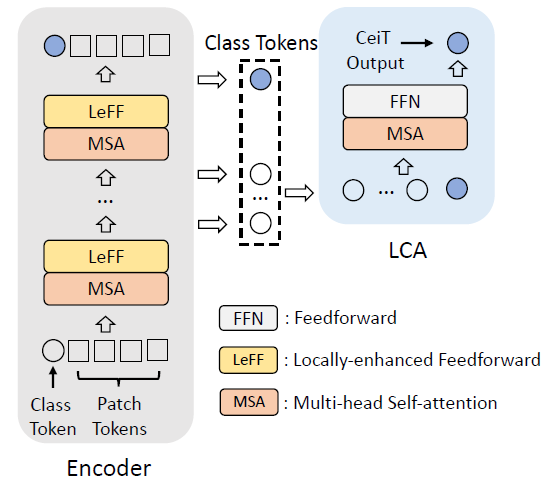I2T模块和普通分patch方法的复杂度：

$$p\times p\times 3\times \frac{H}{p}\times \frac{W}{p} \times C=3CHW$$

I2T模块先进行一步卷积，卷积的 $k=7,S=2$ ，所以计算量是：

$$7\times 7\times 3\times \frac{H}{2}\times \frac{W}{2} \times D=\frac{147}{4}DHW\$$

$$3\times 3\times \frac{H}{4}\times \frac{W}{4} \times D=\frac{9}{16}DHW\$$

$$\frac{p}{S}\times \frac{p}{S}\times D\times \frac{H}{p}\times \frac{W}{p} \times C=\frac{1}{16}HWDC\$$

I2T模块的总计算量是： $(\frac{147}{4}+\frac{9}{16})DHW+\frac{1}{16}HWDC$

LeFF和FFN的复杂度：

LCA模块的复杂度：

Experiments：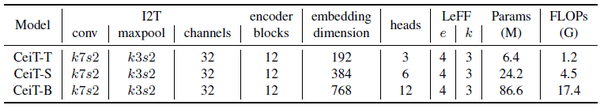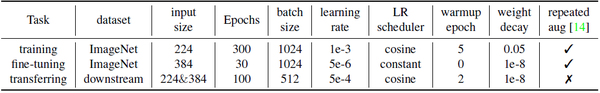ImageNet实验结果：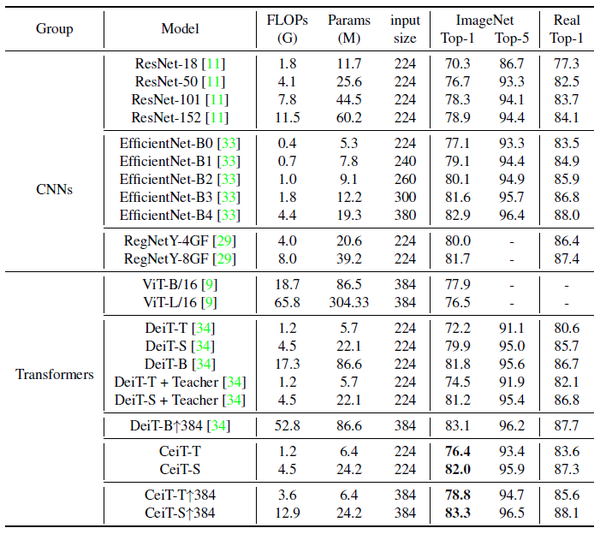CeiT在小数据集上的结果如图21所示。CeiT-S在大多数数据集上都超过了DeiT-B，并具有更小的参数量和计算量。在分辨率为384的数据集上fine-tune之后的CeiT-S模型的性能可以与EfficientNet-b7比较，展示出了将CNN与Transformer模型结合的潜力。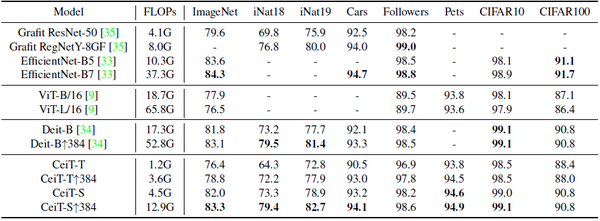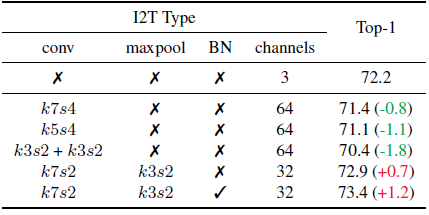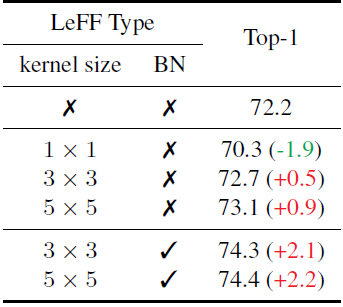CeiT的思路和上一篇CvT极其相似，都是在图像分patch之前先通过一个卷积操作，提取low-level的信息，再进行后续的操作。只是CvT是每个stage来一次卷积，一共3次。CeiT是只在一开始分patch之前来一次这样的操作，一共就1次。另外，都是在Linear Projection操作中融入了卷积，方法都是先把 $(HW,d)$ 的特征reshape成2D的feature，再经过Depth-wise convolution操作，至于为什么是Depth-wise convolution而不是简单的卷积，很显然是为了节约计算量，否则卷积计算量太大导致作者不好讲故事。

• CNN和Transformer结合是一个涨点的方向，可以发挥2者的优势。
• Linear Projection操作可以转化成卷积，模型照样会work，这一点2篇文章都已经证明了。
• 图片分patch之前可以先用卷积提特征，这一点2篇文章都已经证明了。
• 这个坑已经被填了，如果后面的研究者再想做个CNN + Transformer的工作，可能要考虑换个角度了。

## Summary：

CvT首先embedding的方式变成了卷积操作，在每个Multi-head self-attention之前都进行Convolutional Token Embedding。其次在 Self-attention的Projection操作不再使用传统的Linear Projection，而是使用Convolutional Projection。最后取消位置编码，因为卷积操作包含了暗位置信息。

CeiT的思路和上一篇CvT极其相似，都是在图像分patch之前先通过一个卷积操作，提取low-level的信息，再进行后续的操作。只是CvT是每个stage来一次卷积，一共3次。CeiT是只在一开始分patch之前来一次这样的操作，一共就1次。另外，都是在Linear Projection操作中融入了卷积，方法都是先把 $(HW,d)$ 的特征reshape成2D的feature，再经过Depth-wise convolution操作。